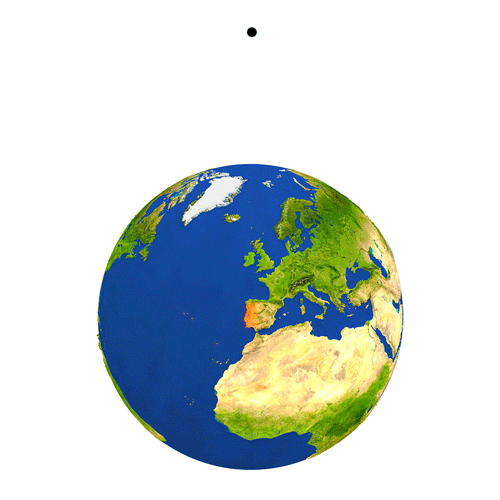# Is It Uniform Acceleration?Assume that Earth is a perfect sphere with radius $R.$ The time $T$ an object takes to fall to the ground from rest from a height of $R$ above the ground is given by $T=\sqrt{\frac Rg}\left(A+\frac {\pi^B}C\right),$ where $A,B,C$ are positive integers.

Find $A+B+C.$

Note: Consider only gravity, and forget about atmospheric effects, air resistance, etc.

×